In this article, we will share MP Board Class 10th Maths Book Solutions Chapter 7 Coordinate Geometry Ex 7.4 Pdf, These solutions are solved subject experts from the latest edition books.

## MP Board Class 10th Maths Solutions Chapter 7 Coordinate Geometry Ex 7.4

Question 1.
Determine the ratio in which the line 2x + y – 4 = 0 divides the line segment joining the points A(2, -2) and 8(3, 7).
Solution:
Let the required ratio be k : 1 and the point C divide them in the above ratio.
∴ Coordinates of C are $$\left(\frac{3 k+2}{k+1}, \frac{7 k-2}{k+1}\right)$$
Since, the point C lies on the given line 2x + y – 4 = 0.
∴ We have $$2\left(\frac{3 k+2}{k+1}\right)+\left(\frac{7 k-2}{k+1}\right)-4$$ = 0
⇒ 2(3k + 2) + (7k – 2) = 4 × (k + 1)
⇒ 6k + 4 + 7k – 4k – 4 – 2 = 0
⇒ (6 + 7 – 4)k + (-2) = 0 ⇒ 9k – 2 = 0
⇒ k = $$\frac{2}{9}$$
The required ratio = k : 1 = $$\frac{2}{9}$$ : 1 = 2 : 9

Question 2.
Find a relation between x and y if the points (x, y), (1, 2) and (7, 0) are collinear.
Solution:
Let the given points be A(x, y), B( 1, 2) and C(7, 0) are collinear.
The points A, B and C will be collinear if area of ∆ABC = 0
⇒ $$\frac{1}{2}$$ [x(2 – 0) + 1(0 – y) + 7(y – 2) = 0
or 2x – y + 7y – 14 = 0
or 2x + 6y – 14 = 0 or x + 3y – 7 = 0, which is the required relation between x and y.Question 3.
Find the centre of a circle passing through the points (6, -6), (3, -7) and (3, 3).
Solution:
Let the points are A (6, -6), B(3, -7) and C(3, 3)
Let P(x, y) be the centre of the circle. Since, the circle is passing through A, B and C.
∴ AP = BP = CP
Taking AP = BP, we have AP2 = BP2
⇒ (x – 6)2 + (y + 6)2 = (x – 3)2 + (y + 7)2
⇒ x2 – 12x + 36 + y2 + 12y + 36 = x2 – 6x + 9 + y2 + 14y + 49
⇒ – 12x + 6x + 12y – 14y + 72 – 58 = 0
⇒ – 6x – 2y + 14 = 0
⇒ 3x + y – 7 = 0 ……………….. (1)
Taking BP = CP, we have BP2 = CP2
⇒ (x – 3)2 + (y + 7)2 = (x – 3)2 + (y – 3)2
⇒ x2 – 6x + 9 + y2 + 14y + 49 = x2 – 6x + 9 + y2 – 6y + 9
⇒ – 6x + 6x + 14y + 6y + 58 -18 = 0
⇒ 20y + 40 = 0
⇒ y = $$\frac{-40}{20}$$ = -2
From (1) and (2), 3x – 2 – 7 = 0
⇒ 3x = 9 ⇒ x = 3
i.e., x = 3 and y = -2
∴ The required centre is (3, -2).

Question 4.
The two opposite vertices of a square are (-1, 2) and (3, 2). Find the coordinates of the other two vertices.
Solution:
Let we have a square ABCD such that A(-1, 2) and C(3, 2) are the opposite vertices. Let B(x, y) be an unknown vertex.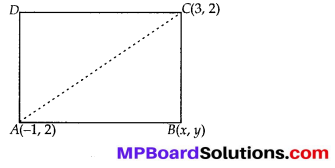Since, all sides of a square are equal.
∴ AB = BC ⇒ AB2 = BC22
⇒ (x + 1)2 + (y – 2)2 = (x – 3)2 + (y – 2)2
⇒ x2 + 2x + 1 + y2 – 4y + 4
⇒ x2 – 6x + 9 + y2 – 4y + 4
⇒ 2x + 1 = -6x + 9
⇒ 8x = 8 ⇒ x = 1  …………………. (1)
Since, each angle of a square = 90°.
∴ ∆ABC is a right angled triangle.
∴ Using Pythagoras theorem, we have AB2 + BC2 = AC2
⇒ (x + 1)2 + (y – 2)2] + [(x – 3)2 + (y – 2)2]
= [(3 + 1)2 + (2 – 2)2]
⇒ [x2 + 2x + 1 + y2 – 4y + 4] + [x2 – 6x + 9 + y2 – 4y + 4]
= [42 + 02]
⇒ 2x2 + 2y2 + 2x – 4y – 6x – 4y + 1 + 4 + 9 + 4 = 16
⇒ 2x2 + 2y2 – 4x – 8y + 2 = 0
⇒ x2 + y2 – 2x – 4y + 1 = 0 …………….. (2)
Substituting the value of x from (1) into (2), we have
1 + y2 – 2 – 4y + 1 = 0
⇒ y2 – 4y + 2 – 2 = 0
⇒ y2 – y = 0
⇒ y(y – 4) = 0
⇒ y = 0 or y = 4
Hence, the required other two vertices are (1, 0) and (1, 4).

Question 5.
The class X students of a secondary school in Krishinagar have been allotted a rectangular plot of land for their gardening activity. Sapling of Gulmohar are planted on the boundary at a distance of 1 m from each other. There is a triangular grassy lawn in the plot as shown in the figure. The students are to sow seeds of flowering plants on the remaining area of the plot.
(i) Taking A as origin, find the coordinates of the vertices of the triangle.
(ii) What will be the coordinates of the vertices of ∆PQR if C is the origin?
Also calculate the areas of the triangles in these cases. What do you observe?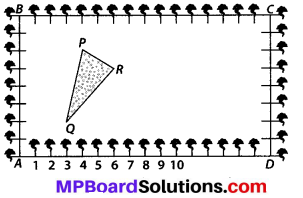Solution:
(i) By taking A as the origin and AD and AB as the coordinate axes. We have P(4, 6), Q( 3, 2) and R( 6, 5) as the vertices of ∆PQR.
(ii) By taking C as the origin and CB and CD as the coordinate axes, then the vertices of ∆PQR are P(-12, – 2), Q(-13, – 6) and R(- 10, – 3)
Case I: When P(4, 6), Q(3, 2) and R(6, 5) are the vertices.
∴ ar(∆PQR) = $$\frac{1}{2}$$ [4(2 – 5) + 3(5 – 6) + 6(6 – 2)]
= $$\frac{1}{2}$$ [-12 – 3 + 24] = $$\frac{9}{2}$$ sq. units
Case II: When P(-12, -2), Q(-13, -6) and R(-10, -3) are the vertices.
∴ ar(∆PQR)
= $$\frac{1}{2}$$ [-12(- 6 + 3) + (-13)(- 3 + 2) + (-10)(-2 + 6)]
= $$\frac{1}{2}$$ [-12(-3) + (-13)(-1) + (-10) × (4)]
= $$\frac{1}{2}$$ [36 + 13 – 40] = $$\frac{9}{2}$$ sq. units
Thus, in both cases, the area of ∆PQR is the same.Question 6.
The vertices of a ∆ABC are A(4, 6), B(1, 5) and C(7, 2). A line is drawn to intersect sides AB and AC at D and E respectively, such that $$\frac{A D}{A B}=\frac{A E}{A C}=\frac{1}{4}$$ Calculate the area of the ∆ADE and compare it with the area of ∆ABC. [Recall “The converse of basis proportionality theorem”, and “theorem of similar triangles taking their areas and corresponding sides”]
Solution: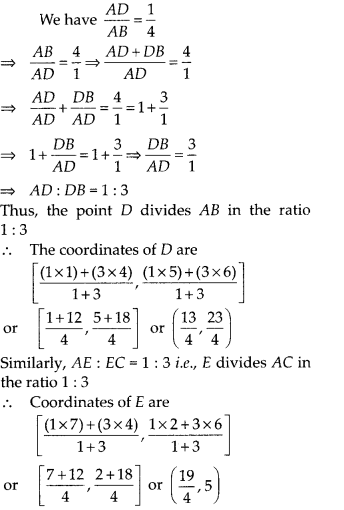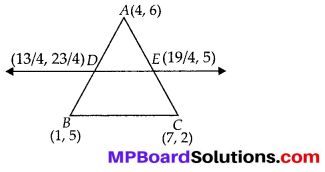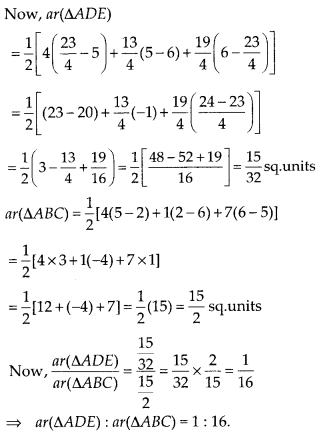Question 7.
Let A(4, 2), B(6, 5) and C(1, 4) be the vertices of ∆ABC.
(i) The median from A meets BCat D. Find the coordinates of the point D.
(ii) Find the coordinates of the point P on AD such that AP : PD = 2 : 1.
(iii) Find the coordinates of points Q and R on medians BE and CF respectively such that BQ : QE = 2 : 1 and CR : RF = 2 : 1
(iv) What do you observe?
[Note : The point which is common to all the three medians is called the centroid and this point divides each median in the ratio 2:1.]
(v) If A(x1, y1), B(x2, y2) and C(x3, y3) are the vertices of ∆ABC, find the coordinates of the centroid of the triangle.
Solution:
We have the vertices of ∆ABC as A (4, 2), B(6, 5) and C(1, 4).
(i) Since AD is a median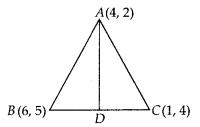∴ Coordinates of D are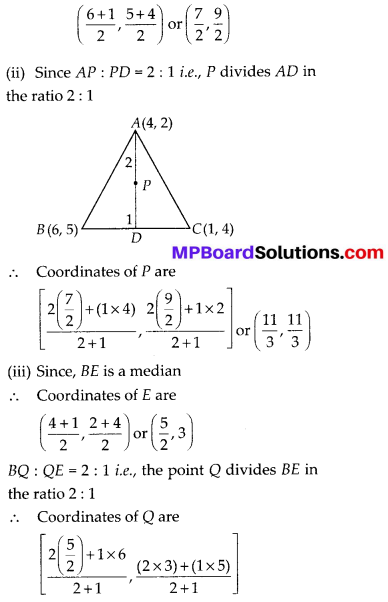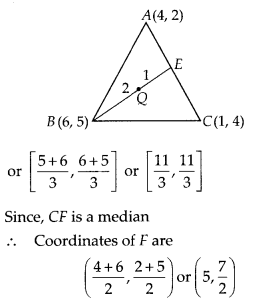Also, CR : RF = 2 : 1 i.e., the point R divides CF in the ratio 2 : 1
∴ Coordinates of R are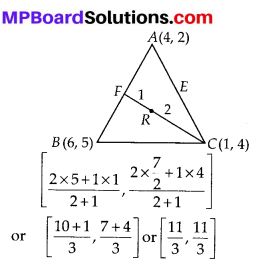(iv) We observe that P, Q and R represent the same point.
(v) Here, we have A(x1, y1), B(x2, y2) and C(x3, y3) are the vertices of ∆ABC. Also AD, BE and CF are its medians.
∴ D, E and F are the mid points of BC, CA and AB respectively.
We know, the centroid is a point on a median, dividing it in the ratio 2 : 1.
Considering the median AD, coordinates of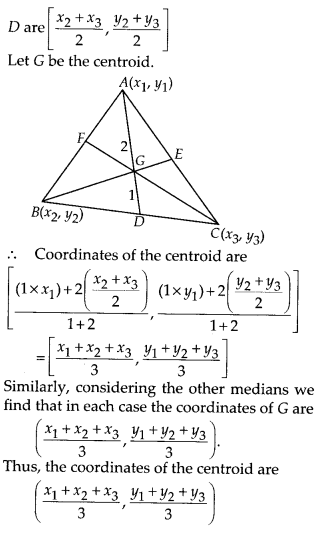Question 8.
ABCD is a rectangle formed by the points A(-1, -1), B(-1, 4), C(5, 4) and D(5, -1). P, Q, R and S are the mid-points of AB, BC, CD and DA respectively. Is the quadrilateral PQRS a square? a rectangle? or a rhombus? Justify your answer.
Solution:
We have a rectangle whose vertices are A(-1, -1), B(-1, 4), C(5, 4) and D(5, -1).
∵ P is mid-point of AB
∴ Coordinates of P are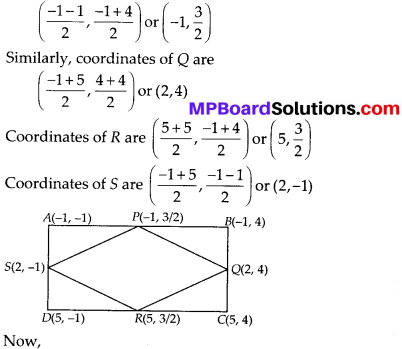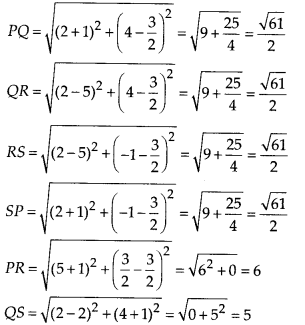We see that PQ = QR = RS = SP i.e., all sides of PQRS are equal.
∴ It can be a square or a rhombus.
But PR ≠ QS i.e., its diagonals are not equal.
∴ PQRS is a rhombus.